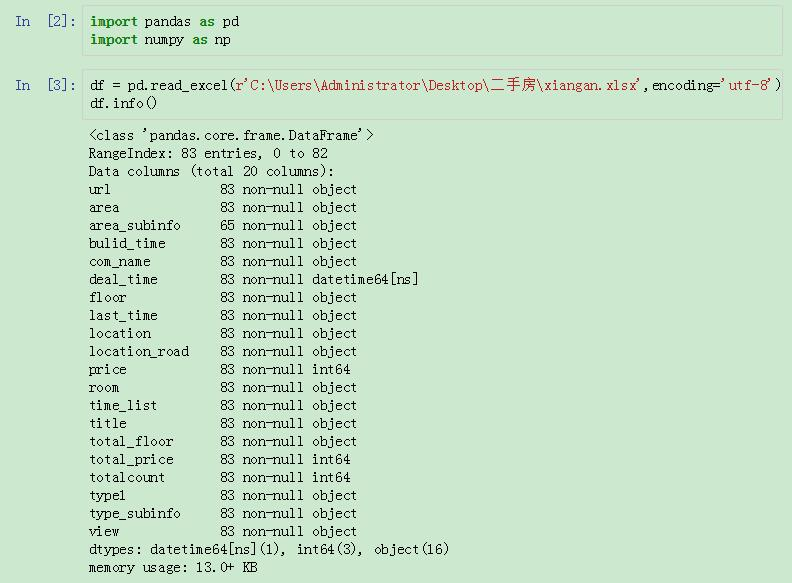# pandas 實現分組後取第N行

## 目的：`solution`

```dfa=df.groupby('question_id').nth(0).reset_index()
dfa['flag']='A'
dfb=df.groupby('question_id').nth(1).reset_index()
dfb['flag']='B'
dfc=df.groupby('question_id').nth(2).reset_index()
dfc['flag']='C'
dfd=df.groupby('question_id').nth(3).reset_index()
dfd['flag']='D'

resdf=dfa.append([dfb,dfc,dfd])
resdf.sort_values(by='question_id')
```

`result``focus`

```g.nth(0)
#同
g.first()
g.head(1)
g.last()

g.nth(2)
g.nth(-1)

g.nth(0,dropna='any')
g.B.nth(0,dropna='all')
g.groups
g.get_group(134429)
g.discribe()
g.agg([np.mean,np.sum.np,std])
```## 我們來看看單價排在前十的數據：## 單價排在前十的數據

nlargest()的第一個參數就是截取的行數。第二個參數就是依據的列名。## 按照total_price排名

nlargest還有一個參數，keep=’first’或者’last’。當出現重復值的時候，keep=’first’,會選取在原始DataFrame裡排在前面的，keep=’last’則去排後面的。## 先設置一個輔助列## 使用max()## 選取多個指標的TOP(N)

nlargest()函數在這種場景下使用是非常方便的，而且結果也已經默認排好順序瞭。## 分組之後進行求和